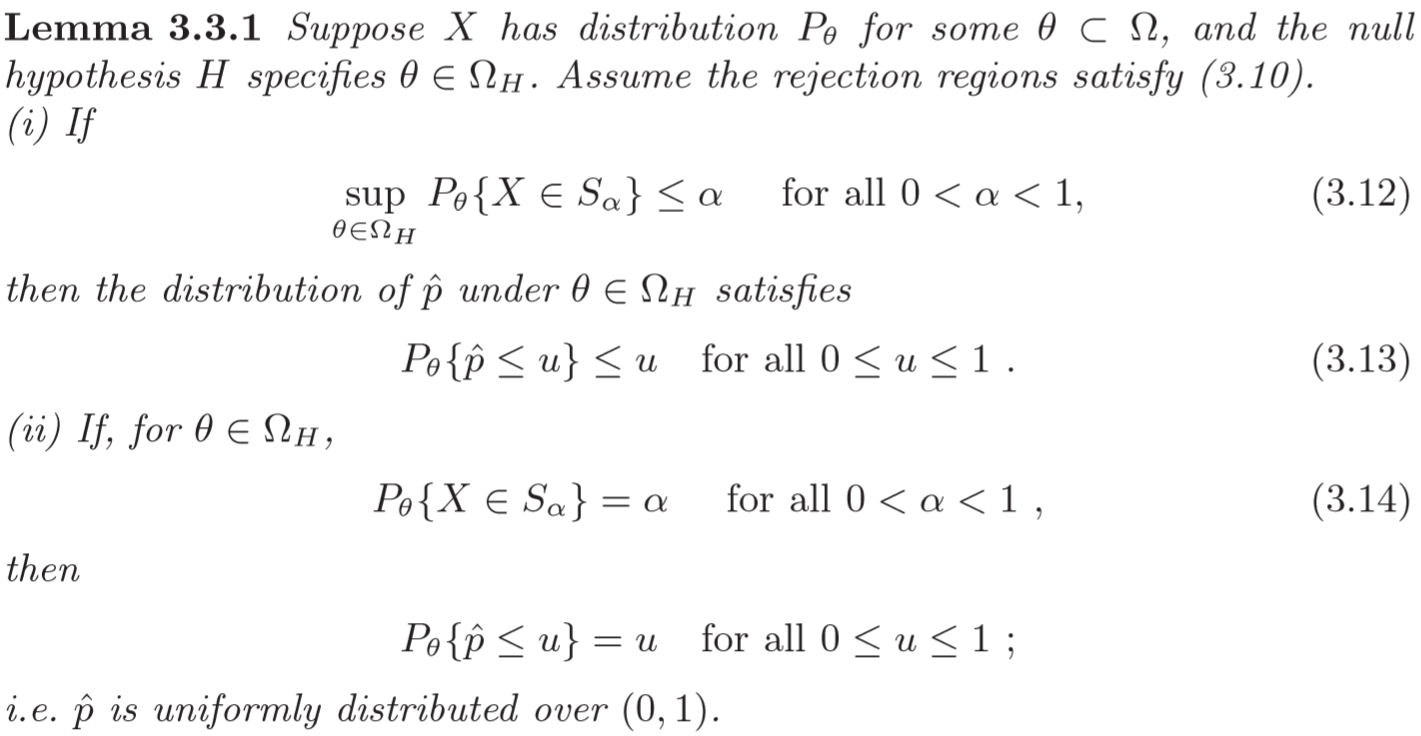# An Adaptive Algorithm for online FDR

##### Posted on Jul 21, 2019

Online false discovery rate (FDR) problem: - observes a possibly infinite sequence of $p$-values $P_1,P_2,\ldots$ - pick a sequence of rejection thresholds $\alpha_1,\alpha_2,\ldots$ in an online fashion

Propose a new framework: SAFFRON - like older alpha-inversting (AI) algorithms, starts off with an error budget, called alpha-wealth, that is intelligently allocates to different tests over time, earning back some wealth on making a new discovery - unlike old methods, the threshold sequence is based on a novel estimate of the alpha fraction that it allocates to true null hypotheses. - it can be seen as an online analogue of the famous offline Storey-BH adaptive procedure. - a monotone version of the original AI algorithm is recovered as a special case of SAFFRON.

## Introduction

The central difficulty when testing a large number of null hypotheses is that several of them may appear to be false, purely by chance.

We would like the set of rejected null hypotheses $\cR$ to have high precision, but separately controlling the false positive rate (type I error) for each individual test actually does not provide any guarantee on the precision.

The false discovery rate (FDR) can provide guarantees on an error metric,

$\FDR \equiv \bbE[\FDP(\cR)] = \bbE\left[ \frac{\vert \cH^0\cap \cR\vert}{\vert \cR\vert} \right]\,.$

Offline multiple testing algorithms take a set of $p$-values ${P_i}$ as their input, and a target FDR level $\alpha\in (0,1)$, and produce a rejected set $\cR$ that is guaranteed to have $\FDR\le \alpha$.

Of course, one also desires a high recall (1- true positive rate), or equivalently a low false negative rate, but without assumptions on many uncontrollable factors like the frequency and strength of signals, additional guarantees on the recall are impossible.

The online problem is emerging as a major area of its own. For example, large information technology companies run thousands of A/B tests every week of the year, and decisions about whether or not to reject the correpsonding null hypothesis must be made without knowing the outcomes of future tests.

Methods:

• first one: alpha-investing algorithm
• generalized alpha-investing algorithm (GAI)
• variants of GAI that control the FDR (as opposed to the modified FDR controlled in the original paper), including a new algorithm called LORD
• GAI++
• LORD++

The paper’s central contribution: the derivation and analysis of a powerful new class of online FDR algorithms called “SAFFRON” (Serial estimate on the Alpha Fraction that is Futilely Rationed On true Null hypotheses)

• as an instance of the GAI framework, it starts off with an error budget, referred to as alpha-wealth, that is allocates to different tests over time, earning back some alpha-wealth whenever it makes a new discovery
• unlike earlier work in the online setting, SAFFRON is an adaptive method.

## Deriving the SAFFRON algorithm

By definition of a $p$-value, if the hypothesis $H_i$ is truly null, then the corrsponding $p$-value is stochastically larger than the uniform distribution (“super-uniformly distributed”), meaning that

If the null hypothesis $H_i$ is true, then $\Pr{P_i\le u}\le u$ for all $u\in[0,1]$.$\cR(t)$: the rejected set after $t$ steps, consists of all $p$-values among the first $t$ ones for which the indicator for rejection is equal to 1. $R_j = 1{P_j\le\alpha_j}$.

Someone considered a modified FDR,

$\text{mFDR}(t) = \frac{\bbE[\vert \cH^0\cap \cR(t)\vert]}{\bbE[\vert \cR(t)\vert]}$
• $\cF^t:=\sigma(R_1,\ldots,R_t)$
• $\alpha_t:=f_t(R_1,\ldots,R_{t-1})$

the null $p$-values are conditionally super-uniformly distributed if the following holds:

If the null hypothesis $H_i$ is true, then $\Pr{P_t\le\alpha_t\mid \cF^{t-1}}\le\alpha_t$.

### An oracle estimate of the FDP and a naive overestimate

Define an oracle estimate of the FDP as

$\FDP^*(t) = \frac{\sum_{j\le t,j\in\cH^0}\alpha_j}{\vert \cR(t)\vert}\,.$

Intuitively (???), the numerator overestimates the number of false discoveries, and $\FDP^*(t)$ overestimates the FDP.

One natural way to convert $\FDP^*(t)$ to a truly empirical overestimate of $\FDP(t)$ is to define:

$\widehat\FDP_{\mathrm{LORD}(t)} = \frac{\sum_{j\le t}\alpha_j}{\vert \cR(t)\vert}\,.$

### A better estimate of the alpha-wealth spent on testing nulls

Knowing that we would expect non-nulls to typically have smaller $p$-values, we propose the following novel estimator:

$\widehat\FDP_{\mathrm{SAFFRON}}(t)\equiv \widehat \FDP_\lambda(t) :=\frac{\sum_{j\le t}\alpha_j\frac{1\{P_j>\lambda_j\}}{1-\lambda_j}}{\vert \cR(t)\vert}$

Some intuition: $\frac{1{P_j>\lambda_j}}{1-\lambda_j}$ has a unit expectation whenever $P_j$ is uniformly distributed (null), but would typically havea much smaller expectation whenever $P_j$ is stochastically much smaller than uniform (non-null).

### The SAFFRON algorithm for constant $\lambda$

In words, SAFFRON starts off with an alpha-wealth, never loses wealth when testing candidate $p$-values, gain wealth of $(1-\lambda)\alpha$ on every rejection except the first.

Published in categories Memo